# The geometry of the fourth dimension and the space-time

on

In Physics, a dimension can be defined as the magnitude of something in a particular direction. Everything that surrounds us is made up of three dimensions.

These are known as three-dimensional or 3D objects. Before we look into the fourth dimension, first we need to understand the first three dimensions.

## The zero dimension

For example, let’s take a sheet of paper. Place a dot on the paper. A dot does not have a direction or a magnitude. So, we can say that this dot does not have any dimensions. Let’s call this a zero dimension (0D).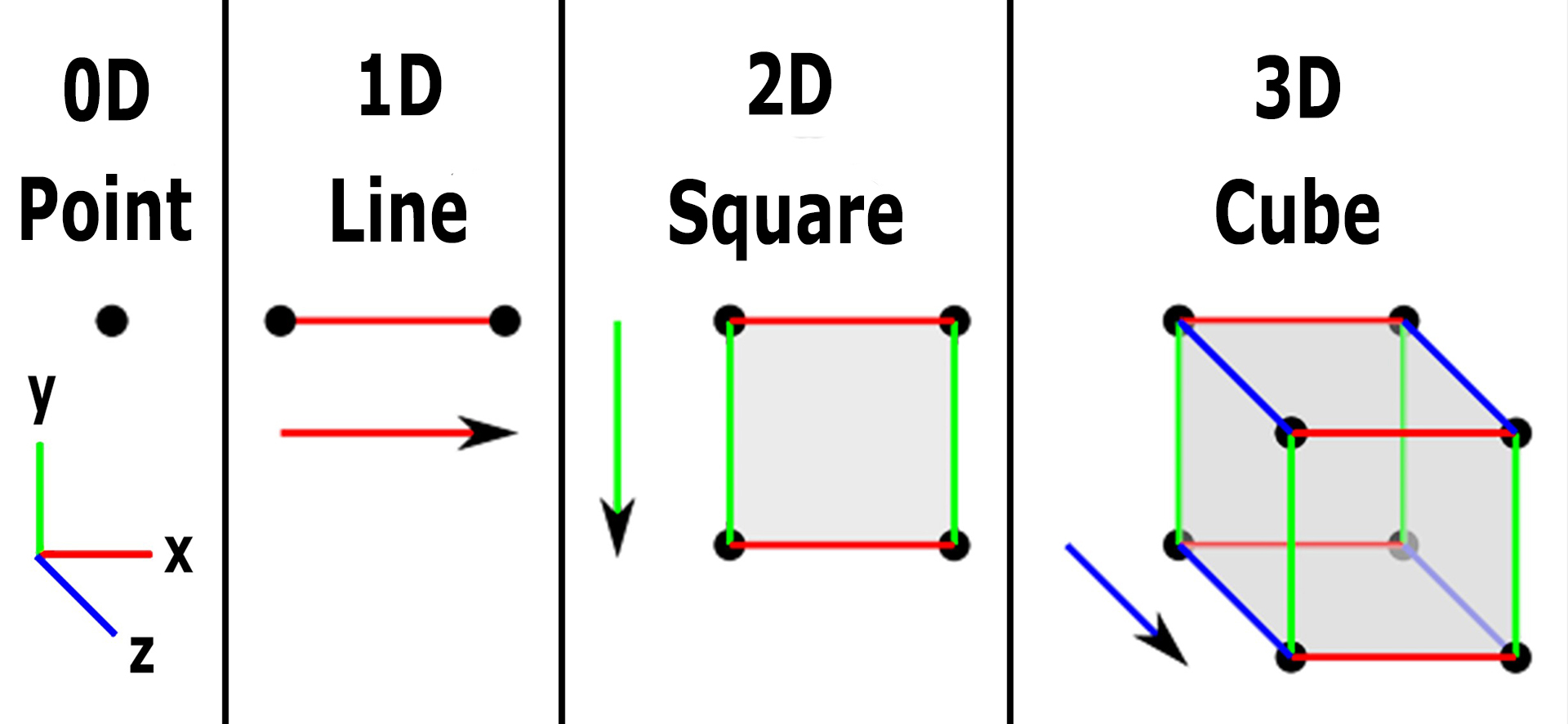0D, 1D, 2D, and 3D.

## The first dimension

Next, from this dot, let’s draw a straight line along the x-axis. Now, we can measure the length of this line. So, we can say that this line has only one dimension. This is called a one-dimensional object or 1D.

## The second dimension

Next, from this line, draw lines on both ends along the y-axis and convert this into a square. Now, for this square, we can measure both its length and width. As we are able to measure both it’s length and width, now we can say that this square has two dimensions. These are called two-dimensional or 2D objects.

## The third dimension

Next, from this square, draw lines along the z-axis and convert this into a cube. Now, we can measure not only its length and width but also its height. As we can measure its length, width, and height, now we can say that this cube has three dimensions. These are called three-dimensional or 3D objects.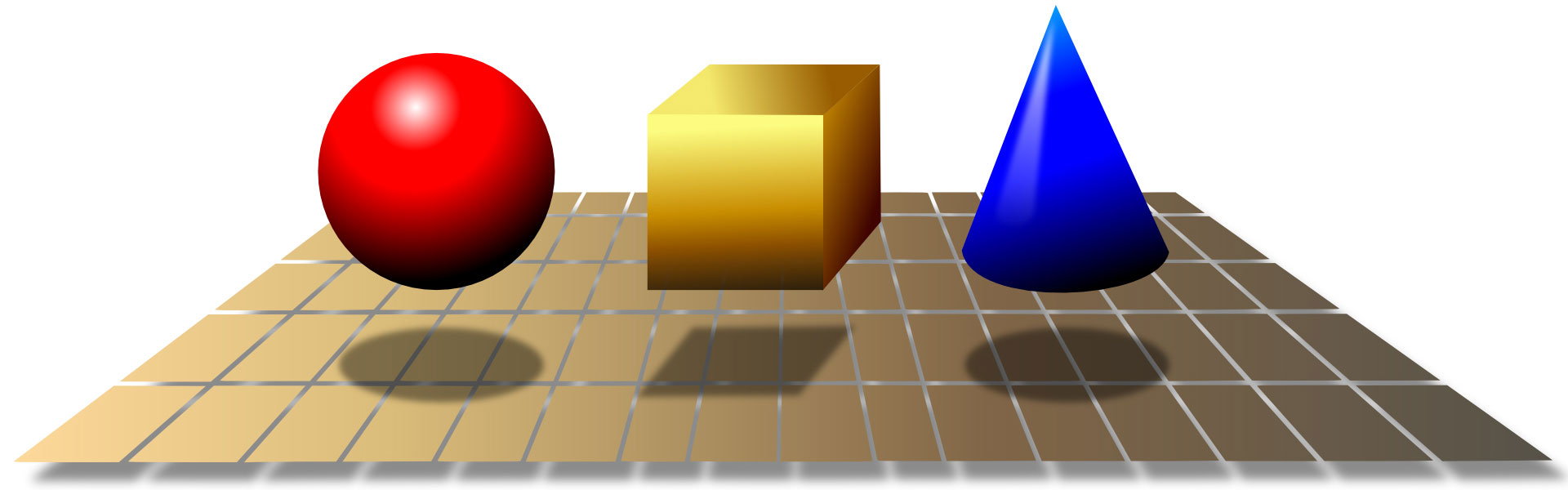3D objects

Similarly, objects that we see around us have length, width, and height making them three-dimensional.

## The fourth dimension and the space-time continuum

In 1905, Albert Einstein published his Special theory of relativity which considers time as the fourth dimension.

Let’s take a look at the cube again. As long as the cube is stationery, we can pinpoint its position along x,y and z-axis.

But, what if this cube starts moving? How can we pinpoint its exact position?

In order to say the position of this moving object, Einstein introduced a dimension called “Time” into this 3D space.

You may wonder how can “Time” be a dimension? For something to be a dimension, it must have a direction and a magnitude. Similarly, time can be measured (ex: seconds).

And, time always flows in the forward direction. So, Einstein considered time as the fourth dimension. Now, with the help of time, we can pinpoint the exact location of this moving cube.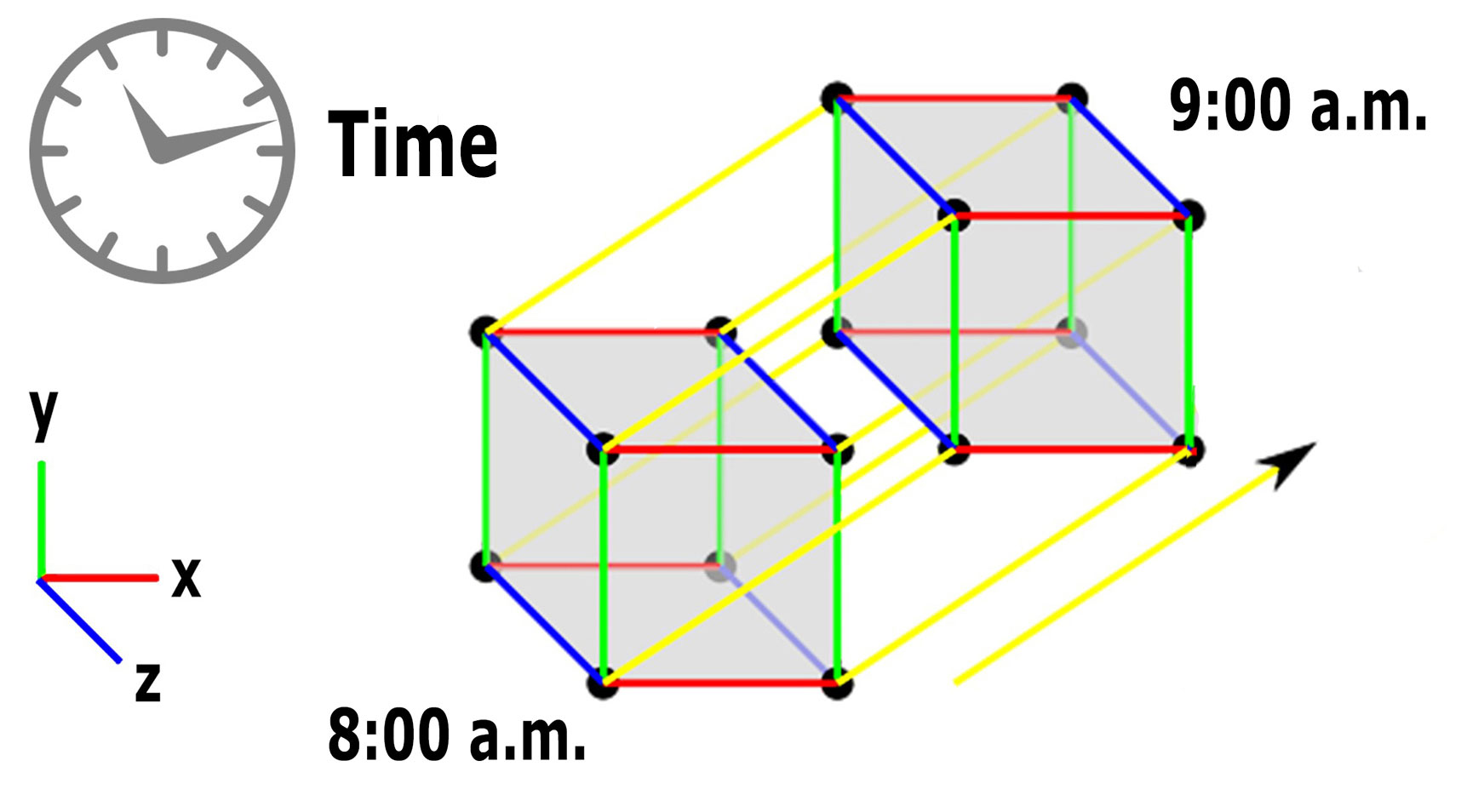Time as the fourth dimension.

For example, we can say that at 8:00 am this cube was at a particular downward position (x,y,z) and at 9:00 am it was at an upward location (x,y,z) with the help of time.

## The geometry of the fourth dimension

While the first three dimensions including line, square, and cube are all geometries, how can the fourth dimension alone be just “time”? According to Albert Einstein’s space-time continuum, time is the fourth dimension.

But in mathematical geometry, the fourth dimension is a shape. To understand the geometry of the fourth dimension, we need to see the first three dimensions from a different perspective.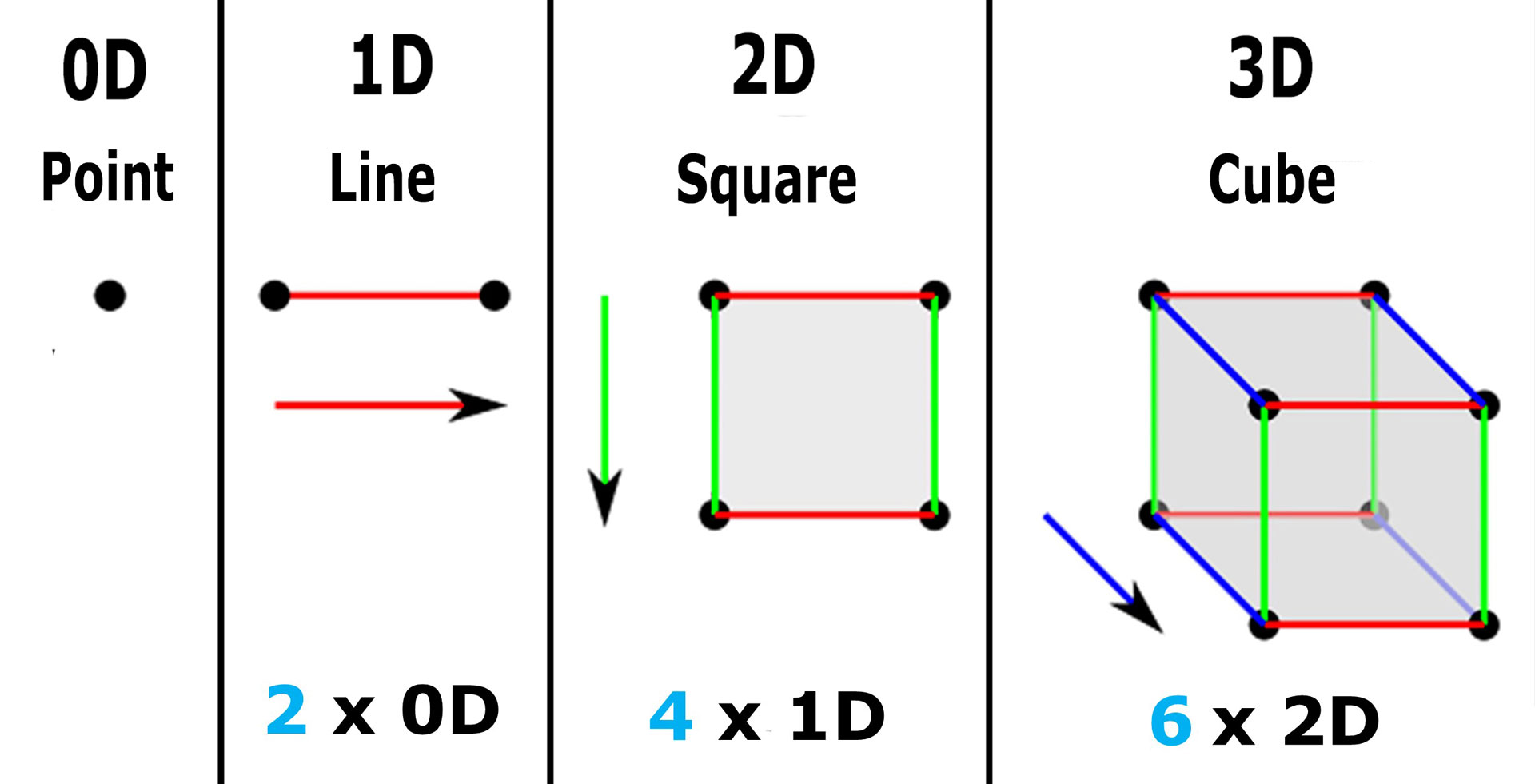Let’s come back to the dot. We know that a dot has no dimensions.

The line that we drew from this dot has 2 dots. A dot at the start of the line and a dot at the end of the line.

The square that we drew from this line has lines on all of its 4 sides.

The cube that we drew from this square has squares on all of its 6 sides.

Let’s say this in a more simple way. The first dimension has 2 zero dimensions. The second dimension has 4 one-dimensions. The third dimension has 6 two dimensions.

If we follow the same order, then the fourth dimension should have 8 cubes.

4D = 8 x 3D

The fourth dimension should have 8 three dimensions. If we draw according to this, this is the shape of the 4th dimension.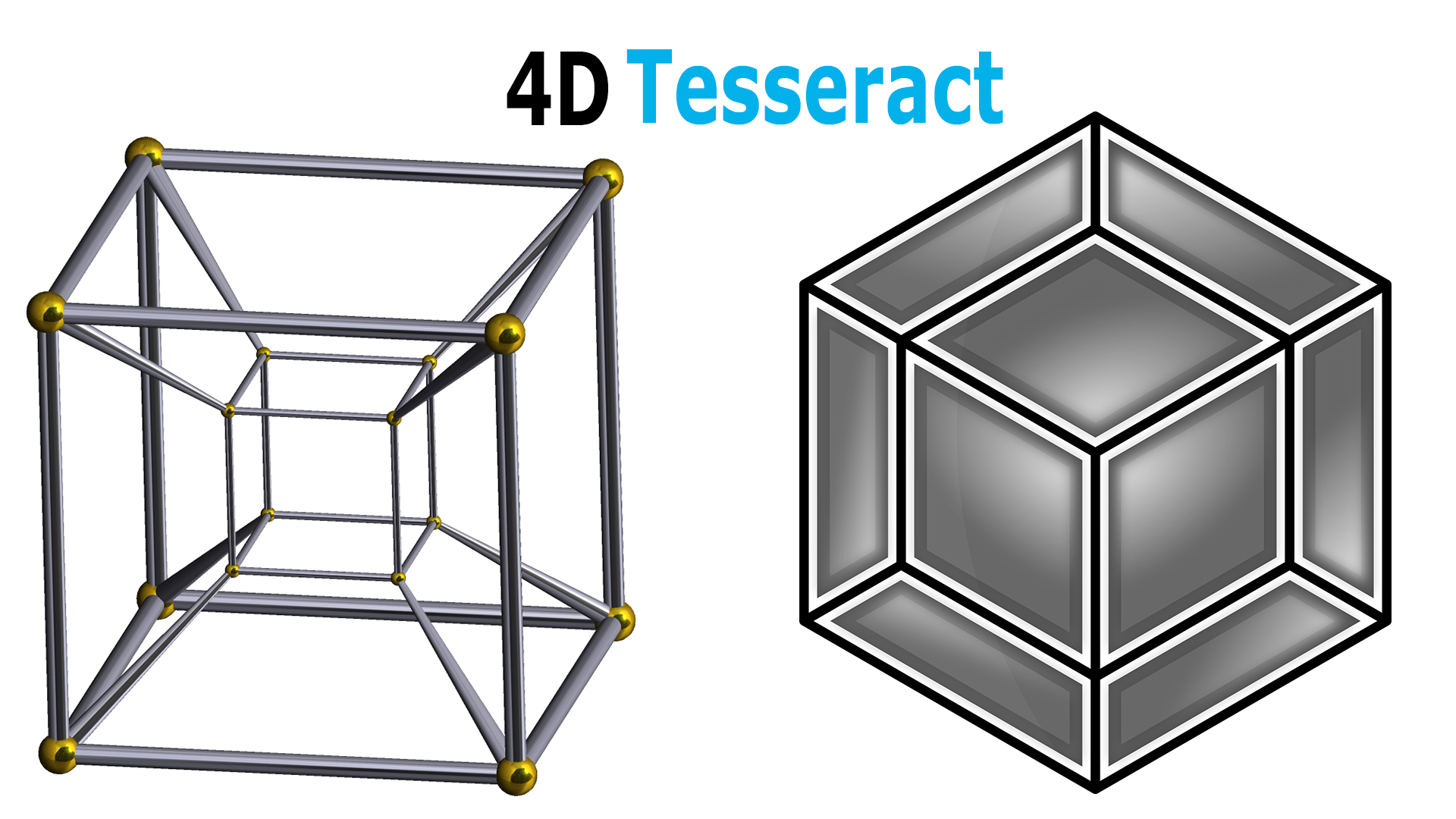This shape is known as Tesseract. A Tesseract has 8 cubes in it. In geometry, as we increase the number of dimensions, their shapes will get more and more complicated to understand using our eyes.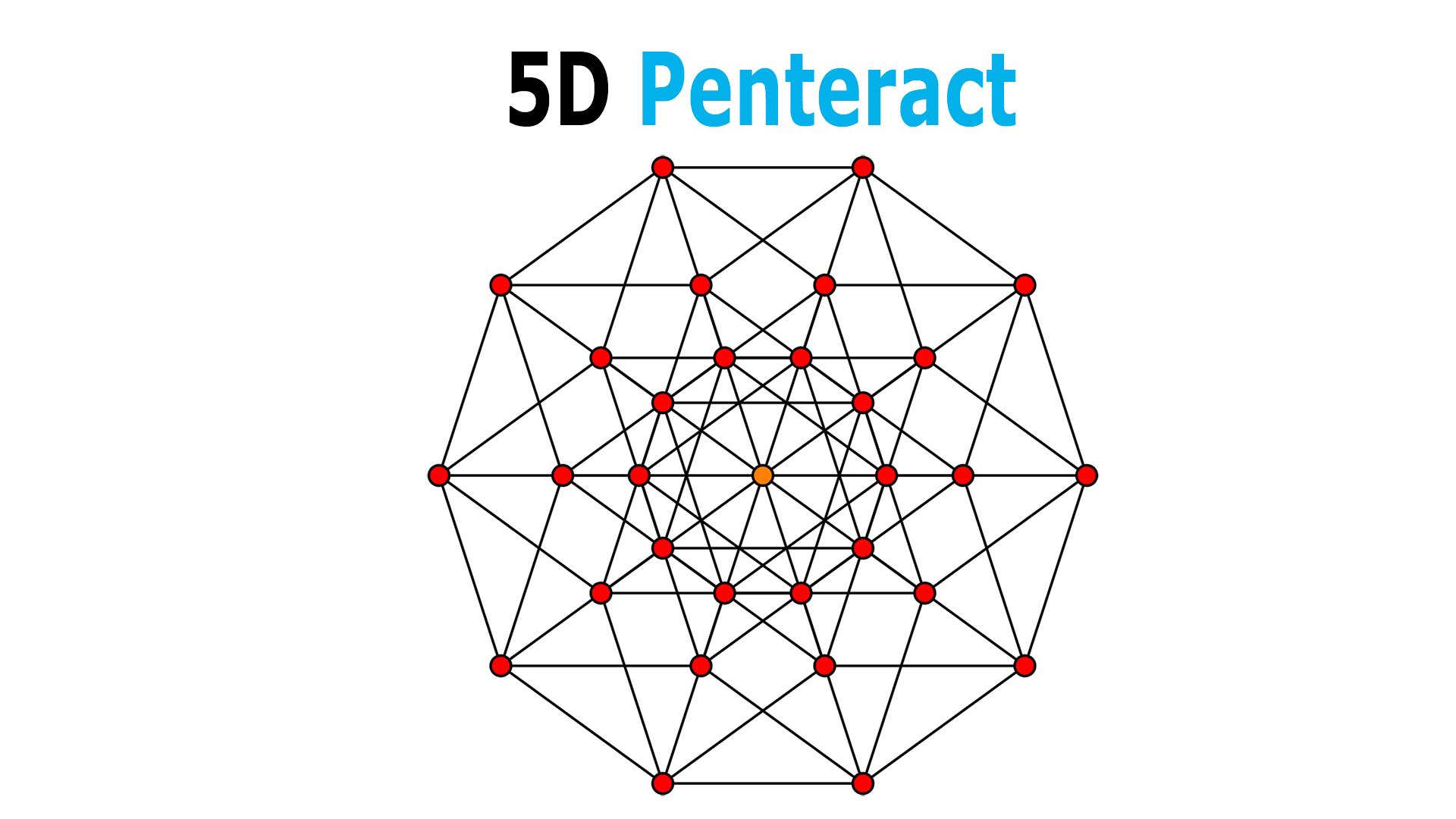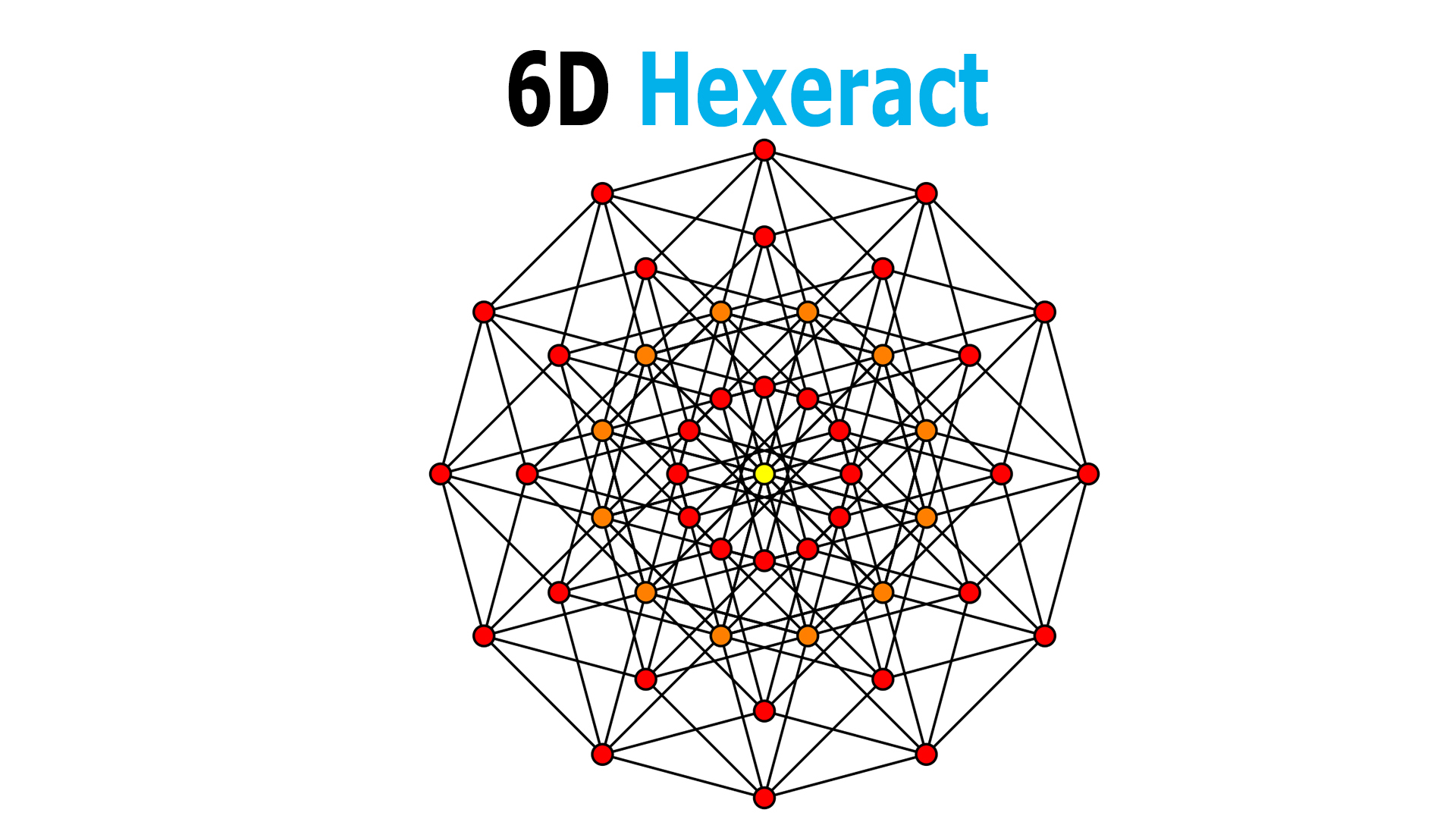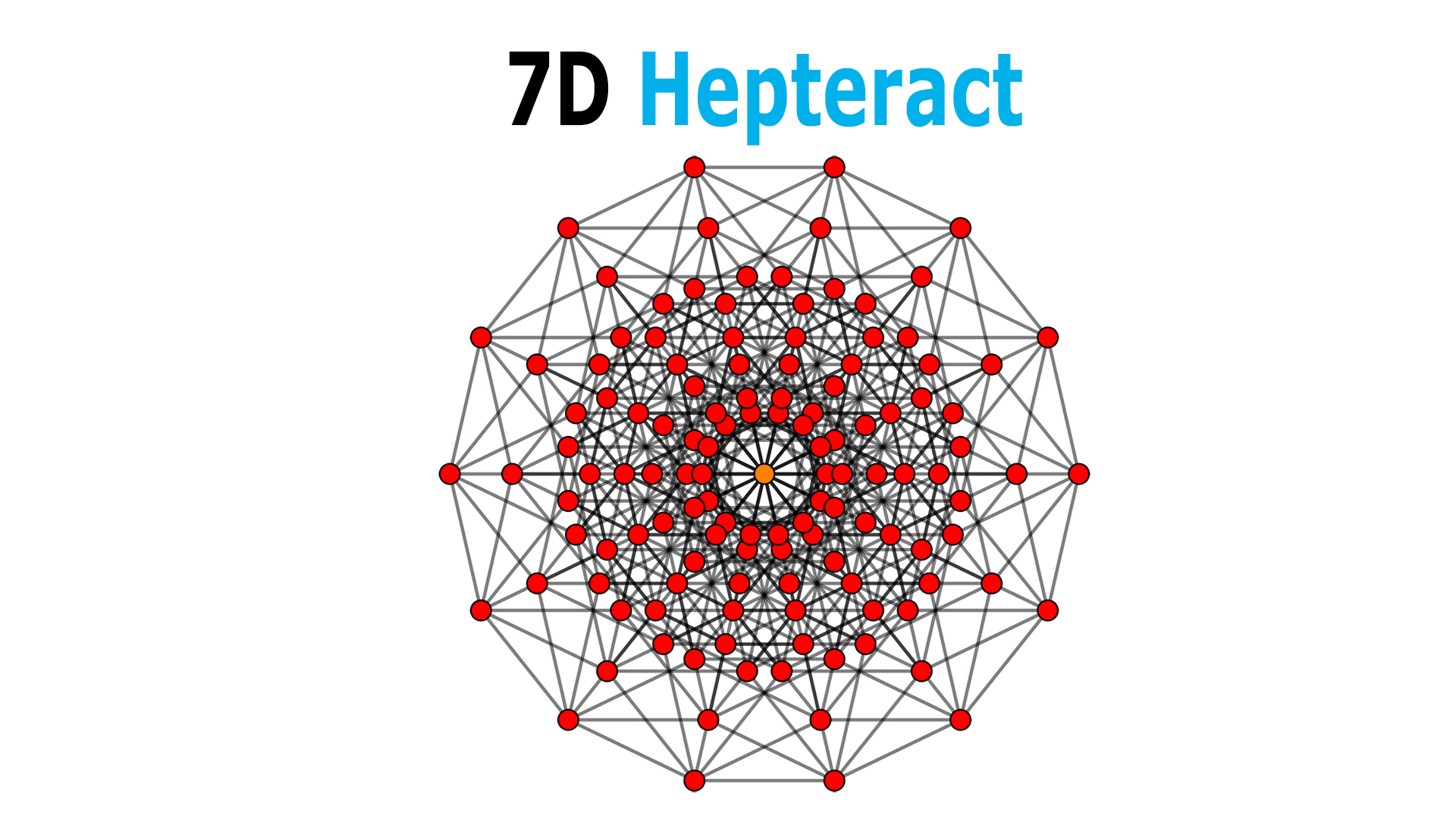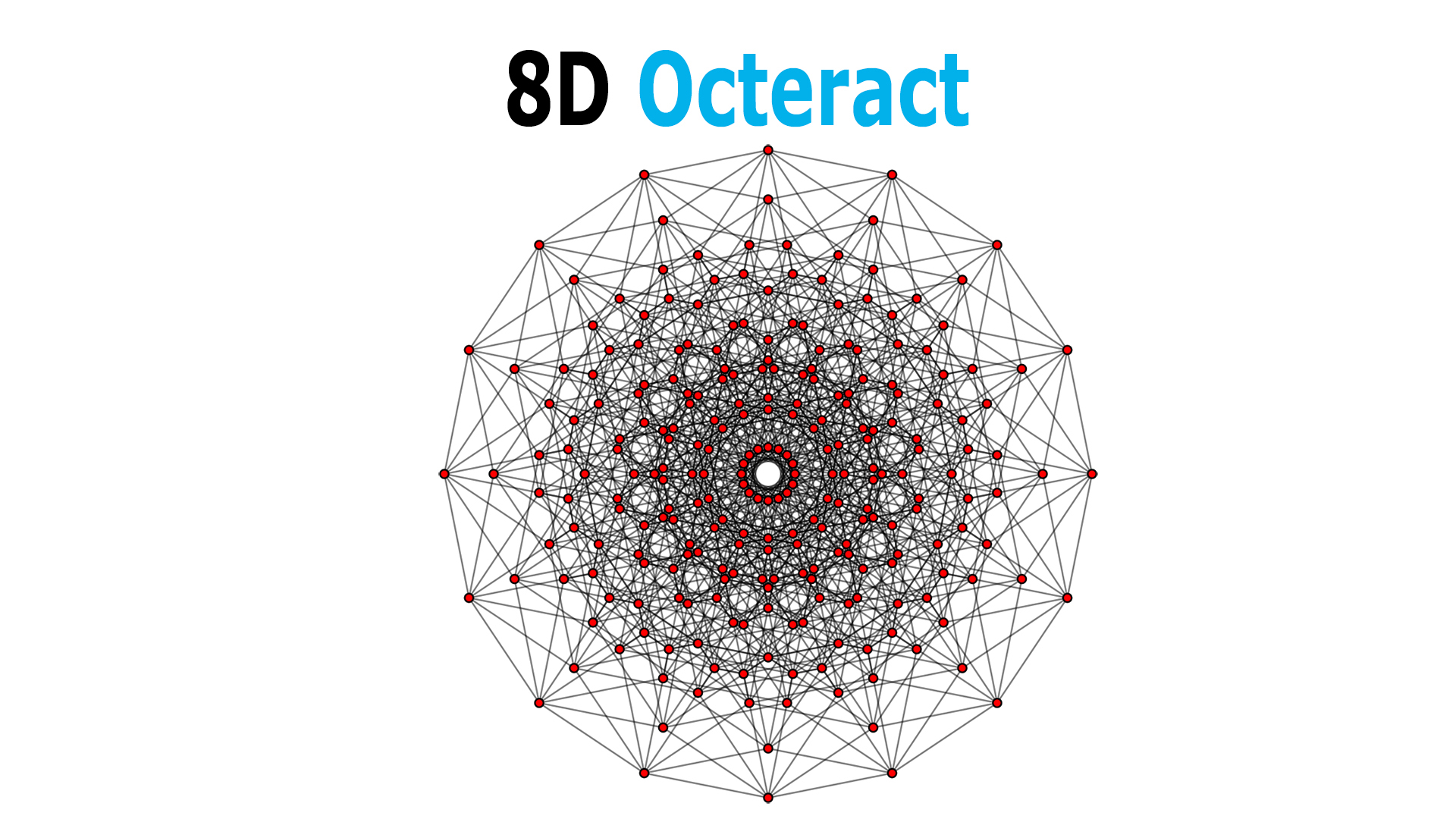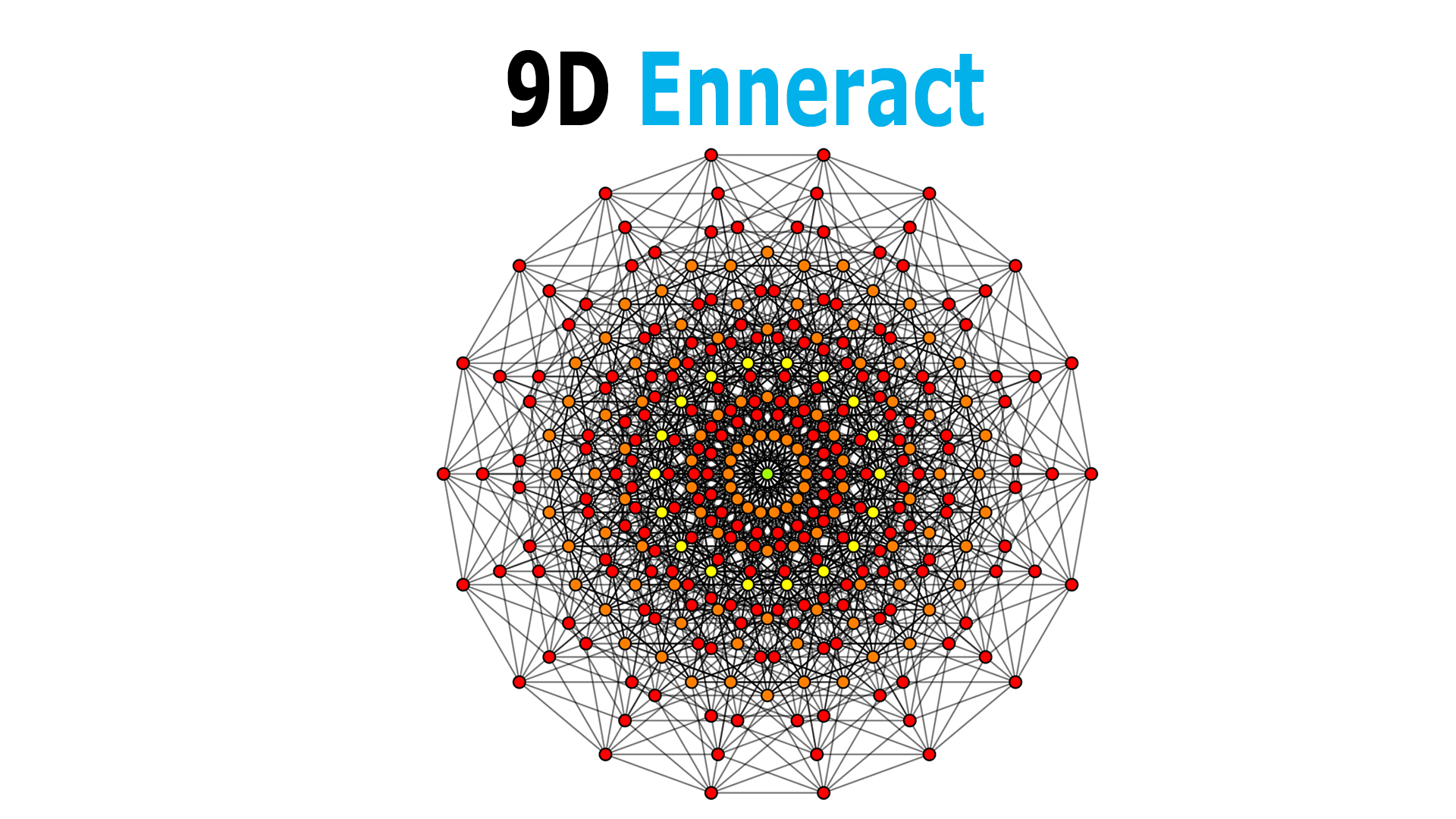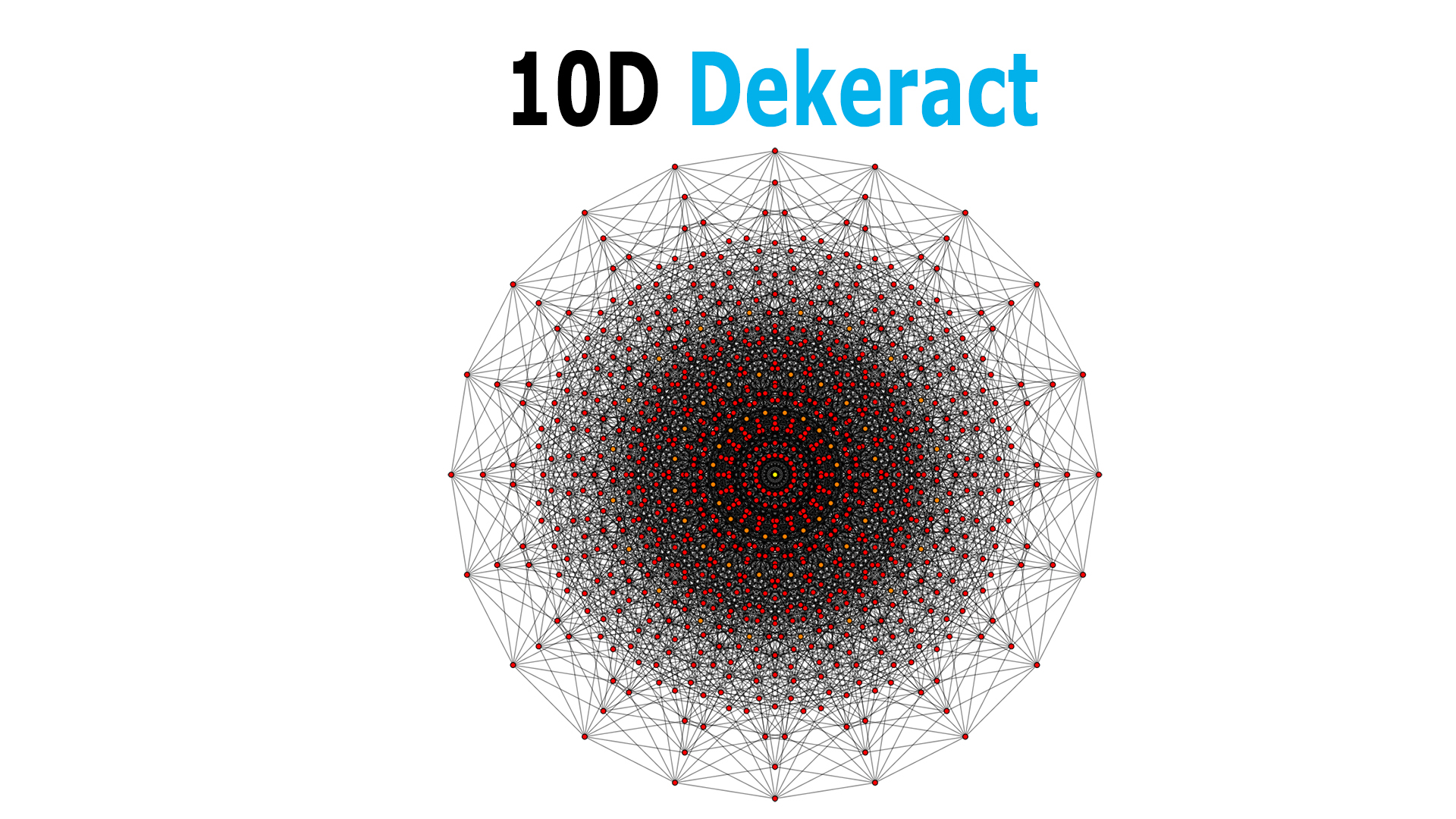Aeronautical engineer, science writer, and the contributing editor of Digitash.

1.fourth dimension

July 27, 2018 at 11:35 AM

In an ordinary three-dimensional world you can not find four-dimensional objects, but you can see them on this YouTube channel.
•